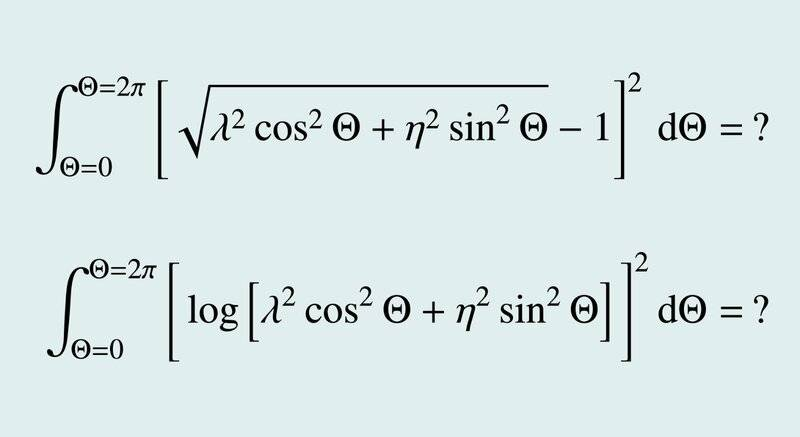# Analytical solution for an integral in polar coordinates?

• A
Summary:
Seeking the solution of an integral over polar coordinates.
Hi,

I am trying to find open-form solutions to the integrals attached below. Lambda and Eta are positive, known constants, smaller than 10 (if it helps). I would appreciate any help! Thank you!anuttarasammyak
Gold Member
As for 1st integral after squared most of the terms are easily integrated except
$$-2 \int \sqrt{\lambda^2 \cos^2\theta + \eta^2 \sin^2\theta} \ d\theta$$
$$=-2\lambda\int \sqrt{1+a\sin^2\theta}\ d\theta$$
where
$$a = \frac{\eta^2-\lambda^2}{\lambda^2}> -1$$
Say ##\lambda > \eta## you may express the integrand as Taylor series terms of which are easily integrated. Say ##\lambda < \eta## you can do similar thing for integrand ##\sqrt{1+b \cos^2\theta}##. Similar Taylor expansion seem to apply for the 2nd integral also.

Last edited:
As for 1st integral after squared most of the terms are easily integrated except
$$-2 \int \sqrt{\lambda^2 \cos^2\theta + \eta^2 \sin^2\theta} \ d\theta$$
$$=-2\lambda\int \sqrt{1+a\sin^2\theta}\ d\theta$$
where
$$a = \frac{\eta^2-\lambda^2}{\lambda^2}> -1$$
Say ##\lambda > \eta## you may express the integrand as Taylor series terms of which are easily integrated. Say ##\lambda < \eta## you can do similar thing for integrand ##\sqrt{1+b \cos^2\theta}##. Similar Taylor expansion seem to apply for the 2nd integral also.
The last integral here looks like a multiple of the complete elliptic integral of the second kind. If a special function counts as an analytical solution, then you might be in luck. As for the logarithmic integral, I'm unclear if you can even find special functions.

•anuttarasammyak